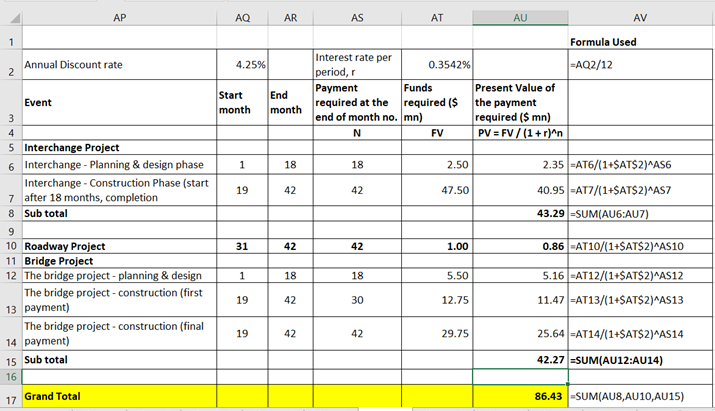Question

Assume that, as a part of its economic development program, your governmental agency has committed to provide access to a new regional industrial park. This project must fund the construction of an on/off-interchange from an adjacent highway, a 2-mile length of 4-lane divided roadway, and a bridge that will cross a 500-foot wide river. The entire project is estimated to require 2 years to complete following planning & design.
A) The interchange is projected to cost \$50 million. It will need to begin construction in 18 months. Rights-of-way acquisition, surveying, and permitting have already been completed and paid for by the program. There will be 2 additional project phases: 1) planning & design, 2) construction. Each will require payment at the end of the phase. The projected cost of the 1st phase of the project is \$2.5 million. The 1st phase is expected to need the entire 18 months prior to the start of construction and must be completed before construction can begin. The 2nd phase is projected to cost the remaining \$47.5 million. It is expected to be completed at the end of the 2-year period following planning & design.
B) The roadway to be constructed is projected to cost \$125,000 per lane mile. It will need to begin construction 12 months prior to the project’s estimated completion date. Your government controls the permitting process for the roadway and has already issued the necessary permits. The total roadway project will be paid for at its completion.
C) At the same time as the interchange is being constructed, the bridge project must also be underway. The bridge is projected to cost \$48 million. It will also need to begin construction in 18 months. Rights-of-way acquisition, surveying, and permitting have already been completed and paid for by the program. There will be 2 additional project phases: 1) planning & design, 2) construction. Each will require payment at the end of the phase. The projected cost of the 1st phase of the project is \$5.5 million. The 1st phase is expected to need the entire 18 months prior to the start of construction and must be completed before construction can begin. The 2nd phase is projected to cost the remaining \$42.5 million. Its construction is also expected to be completed at the end of the 2-year period following planning & design. Unlike the roadway, there will need to be a payout of 30% of the cost of construction at the end of the 1st year of the construction project. The remaining 70% will be paid at the completion of the project.

Assuming a discount rate of 4.25% and monthly compounding, what amount will need to be funded today to meet the complete project’s funding requirements on the payment due dates.

Step 1

Such a problem is better solved in an excel. Hence, I have solved it in excel and provided a snapshot of it along with the excel formula used to calculate the outputs.

But before we get into excel, we need to understand some the facts here:

• The discount rate is 4.25% with monthly compounding. Hence, we need a discount rate perperiod, i.e. per month. Hence, the disocunt rate that will be used for discounting will be discountrate per period = r = discount rate per month = 4.25%/12 = 0.3542%
• The roadway to be constructed is projected to cost \$125,000 per lane mile. It's a 2 mile length, 4 - lane project. Hence, total cost of roadway = \$ 125,000 x 2 x 4 = \$ 1,000,000 = \$ 1 mn
• In the second pahse of bridge project, the first payment occurs at one month after construction. So the maount is 30%. Hence the payment of \$ 42.5 x 30% = \$ 12.75 mn occurs at the end of month no. 30. The balance 70% i.e \$ 42.5 mn x 70% = \$ 29.75 mn occurs at the end of month no. 42.
• All the cash flows given in the question are future values (FVs). We need their present values (PVs). Recall the famour formula: PV = FV x (1 + r)-n
• n is the number of periods i.e. number of months away from today.
Step 2

Please see the table on the white board. Calculations are sequential. All the parts have been calculated one after the other. In the first two coulmn, i have outlined the start and end month. This is to plot the timing of the cash flows. The final answer isshown in the cell highlighted in yellow color. In the last column i have produced the excel formula used in the...

Want to see the full answer?

See Solution

Want to see this answer and more?

Our solutions are written by experts, many with advanced degrees, and available 24/7

See Solution
Tagged in